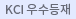#다국어 입력

http://chineseinput.net/에서 pinyin(병음)방식으로 중국어를 변환할 수 있습니다.

변환된 중국어를 복사하여 사용하시면 됩니다.

예시)
• 中文 을 입력하시려면 zhongwen을 입력하시고 space를누르시면됩니다.
• 北京 을 입력하시려면 beijing을 입력하시고 space를 누르시면 됩니다.
닫기

검색결과 좁혀 보기

선택해제
• 좁혀본 항목 보기순서

• 원문유무
• 원문제공처
• 등재정보
• 학술지명
• 주제분류
• 발행연도
• 작성언어
• 저자

오늘 본 자료

• 오늘 본 자료가 없습니다.
더보기
• 무료
• 기관 내 무료
• 유료
•Zone 연신 조건이 PVDF 필름의 압전성에 미치는 영향

Mechanical and piezoelectrical properties of zone-draw poly(vinylidene fluoride) films were studied. Effects of draw ratio and drawing temperature on the mechanical and piezoelectrical behavior were investigated and they were compared with those of hot-drawn films. As draw ratio increases so does the piezoelectric strain constant, but the increase of piezolectric strain constant of zone-drawn films is higher than that of hot-drawn films. Drawing temperature seems to be independent of the piezolectric stain constant. The glass transition temperature determinded by mechanical experiment tends to increase with the increase of draw ratio but that determined by piezoelectrical experiment is independent of draw ratio. This result is the same in both hot and zone-drawn cases.

•Simplified Stress-Strain Relations of Multi-ply Cords

Simplified tensile stress-strain relation of textile cords was derived and discussed with Riding's experimental results. Theoretical equations for two-, three-and seven-ply cords are developed under the consideration of different geometrical packing. The tensile stress-strain relation of cord is expressed in terms of "Cord Factor." As cord twist and cord strain increase, the deviation between theoretical and experimental values increases for the two and three-ply cords. In case of seven-ply cord, the agreement between the calculated and experimental is very good compared with the other two cord structures.

•Effect of Morphology on the Tensile Properties of Poly(ethylene terephthalate) Films

The crystalline, intermediate, and amorphous phase indices were determined by Lindner's X-ray diffraction method and the effect of draw ratio and annealing temperature on the phase indices and the tensile properties were discussed. The intermediate phase increases with draw ratio but slightly decreases with increasing annealing temperature. As the intermediate phase index increases, the initial modulus and breaking stress increase and the breaking strain decreases.

•축차이축연신 Poly(vinylidene Fluoride) 필름의 압전성과 역학적 성질

Piezoelectric and mechanical properties of uniaxially and two step biaxially drawn poly(vinylidene fluoride) films were investigated and discussed in terms of morphology. Piezoelectric constants(e31) of two step biaxially drawn films were higher than those of uniaxially drawn films under the same poling conditions(about 0.5MV/cm.) In two step biaxially drawn films, dynamic tensile moduli in the drawing direction were higher than those in perpendicular direction to the drawing direction. Thermal stability of two step biaxially drawn films were better than that of uniaxially drawn films.

•초고분자량 PE젤 필름과 매트의 Zone 연신 거동

Gel films and mats of ultra-high molecular weight polyethylene were stretched by zone drawing to orient biaxially, and their physical and mechanical properties were studied. The maximum storage modulus of the drawn films at -15$0^{\circ}C$ and room temperature were 215 and 165 GPa, respectively. The drawability of gel film is better than that of gel mat but the maximum storage modulus of gel mat is higher than that of gel film.

•Effect of Zone-Drawing Conditions on the Structure and Physical Properties of Ultrahigh Molecular Weight Polyethylene Single Crystal Mats

Effect of zone-drawing conditions (draw temperature and draw ratio) on the structure and properties of the ultrahigh molecular weight polyethylene(UHMWPE) single crystal mats(SCM) was investigated. Mats were made by slow filtering and drying of single crystals crystallized in the dilute xylene solution of UHMWPE. These mats have platelike lamellar structure with mp 137$^{\circ}C$ and crystallinity 0.71. High draw ratio up to 40 was obtained by zone-drawing of the UHMWPE SCM near melting temperature. The birefringence, crystallinity, tensile modulus and breaking tensile stress of drawn mats appeared not to depend on the draw temperature but increased with increasing draw ratio. The maximum tensile modulus obtained in this experiment was 78 GPa.

•섬유 건조 공정에 있어서 에너지 절약 -건조 온도와 직불두께가 건조시간에 미치는 영향-

The effect of drying temperature and fabric thickness on the drying time was studied on the basis of expermental results. The variations in the amount of free water in the cotton fabric were measured in the drying oven which was maintained at a constant temperature. Mass of free water was measured at various squeezing pressures, drying temperatures, and fabric thicknesses. The theoretical drying time is well coincident with the experimental drying time. Initial moisture regain does not affect the drying rate of cotton fabric when drying temperature is constant. The drying rate of constant drying rate process is proportional to the temperature of air from 85$^{\circ}C$ to 1$25^{\circ}C$. The increase of thickness of fabric does not affect the drying rate of constant drying rate process but makes the critical moisture regain increase. 본 연구는 섬유 공업에 소모되는 에너지의 대부분을 차지하는 건조공정에 대한 연구이며 그 중 특히 건조온도와 직물두께가 건조시간에 미치는 영향에 대해 살펴 보았다. 시료는 잘 정련된 두께 0.343, 0.686 그리고 1.029mm의 면직물을 사용하였으며 각 두께에서 건조온도를 85$^{\circ}C$에서 1$25^{\circ}C$까지 1$0^{\circ}C$씩 변화시키며 실험하였다. 시료의 크기는 22$\times$22($\textrm{cm}^2$)로 하고 각 온도에서 압착 압력을 0.5, 1.0, 1.5, 2.0 그리고 2.5kg/$\textrm{cm}^2$의 5가지로 변화시켜 무게에 따른 건조시간을 측정하였다. 실험 결과로부터 압착압력은 건조온도가 일정할 때 일정건조속도에 영향을 미치지 못하며 일정건조속도는 건조온도($^{\circ}C$)와 일정한 함수관계를 나타내며 직물두께의 증가는 일정건조속도에 변화를 일으키지 않지만 임계점을 수분율이 큰쪽으로 이동시켜 효과적인 건조를 방해함을 알았다. 또한 임계점에서의 수분율은 직물두께의 0.16승에 비계하는 것을 알았다. 본 연구를 통해 초기와 최후의 요망 수분율이 결정되면 각 직물에 따라 효율적인 건조조건의 예측이 가능하다는 것을 알 수 있었다.

해외이동버튼